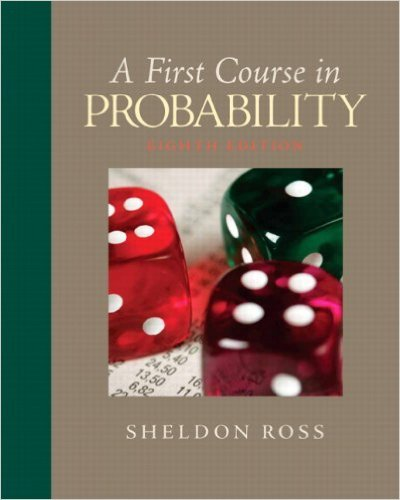×
Get Full Access to First Course In Probability - 8 Edition - Chapter 7 - Problem 7.77
Get Full Access to First Course In Probability - 8 Edition - Chapter 7 - Problem 7.77

×

# The joint density of X and Y is given byf (x, y) = 1ISBN: 9780136033134 163

## Solution for problem 7.77 Chapter 7

First Course in Probability | 8th Edition

• Textbook Solutions
• 2901 Step-by-step solutions solved by professors and subject experts
• Get 24/7 help from StudySoup virtual teaching assistantsFirst Course in Probability | 8th Edition

4 5 1 340 Reviews
14
3
Problem 7.77

The joint density of X and Y is given byf (x, y) = 1 2eye(xy)2/2 0 < y < q,q < x < q (a) Compute the joint moment generating functionof X and Y.(b) Compute the individual moment generatingfunctions.

Step-by-Step Solution:
Step 1 of 3

Color Coding: Topics MINITAB Examples STT 351 Notes Normal −1 2 f x = 1 e 2 x ( ) 2Π ­inf < x < inf √ To find probabilities between two points (a,b] we have to integrate this function between a,b. Using MINITAB: 1) Calc 2) Probability distribution 3) Normal (mean = 0, Standard deviation = 1) 4) Select cumulative probability This will give the area under the curve up to the point x For any a value, the area under the curve up to the point a is = P(X ≤ a) # If we want to find the area under the curve between two points we can use the equation….. P( a < X ≤ b ) = P( X ≤ b ) – P( X ≤ a ) Using MINITAB: To find

Step 2 of 3

Step 3 of 3

##### ISBN: 9780136033134

This textbook survival guide was created for the textbook: First Course in Probability, edition: 8. The answer to “The joint density of X and Y is given byf (x, y) = 1 2eye(xy)2/2 0 < y < q,q < x < q (a) Compute the joint moment generating functionof X and Y.(b) Compute the individual moment generatingfunctions.” is broken down into a number of easy to follow steps, and 39 words. This full solution covers the following key subjects: compute, moment, Joint, generating, functions. This expansive textbook survival guide covers 10 chapters, and 495 solutions. The full step-by-step solution to problem: 7.77 from chapter: 7 was answered by , our top Statistics solution expert on 11/23/17, 05:06AM. Since the solution to 7.77 from 7 chapter was answered, more than 255 students have viewed the full step-by-step answer. First Course in Probability was written by and is associated to the ISBN: 9780136033134.

Unlock Textbook Solution